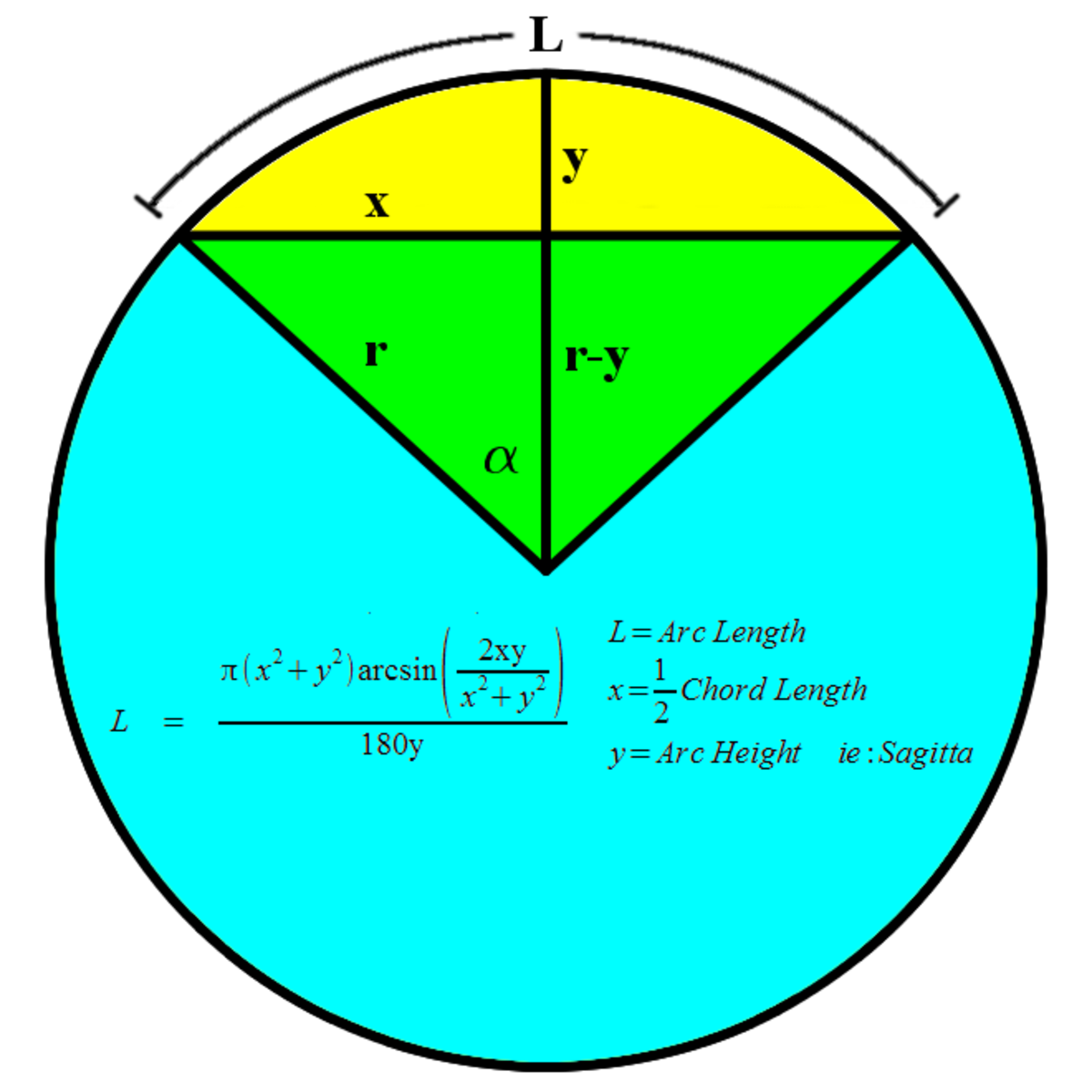# Arc Length Calculation Given Only the Chord Length and Arc Height (Sagitta)

## Arc Length CalculationHow to calculate the length of an arc while only given the chord length and the sagitta or height of the arc at the midpoint perpendicular to the chord .Getting There

## Important Note:

This calculation is only valid when the angle of the arc is less than or equal to 180 degrees. To calculate larger arc lengths, calculate the length of the smaller arc first, then subtract it from the circumference of the circle. This will give you the arc length of the remainder of the circle.

## What application do you have for this calculation?

I for one needed to calculate the heat loss on a greenhouse design I was evaluating. I was deciding between a peaked roof style and a quonset (round roof) style.

I didn't know the radius of the roof curvature or the angle the arc spanned. I did however know the chord length which was the width of the greenhouse and the peak height of the greenhouse. To get the height of the arc, I subtracted the wall height from the peak height, Using the Pythagorean theorem, I was able to solve for the radius of the arc. Then using the law of signs I was able to solve for the angle of the arc.

Since the ratio of the arc length to the circumference of the circle is equal to the ratio of the arc angle to the full angle of the circle (360), I was able to plug the equations I had found for radius and arc angle, into the ratio equation and solve for L.

Now that I was able to calculate the arc length of the roof, I could multiply it by the length of the greenhouse to get the area of the roof that I need for my heat loss calculations

Thank you..

## Arc Length Calculation Derived

getting there (author) on December 10, 2017:

Scroll to Continue

s.b on December 10, 2017:

tank you

getting there (author) on October 12, 2015:

What dimension are you trying to calculate?

mashiq546@yahoo.com on October 12, 2015:

please i have 125 m curve length and 105 m chord length how to calculate do you have any formula for this question.

getting there (author) on January 14, 2014:

Thanks for the feedback paxwill. What sort of curved surfaces are you constructing?

getting there (author) on April 26, 2013:

Thanks for the feedback joanwz. I'm glad you found it useful.

Joan Whetzel on April 26, 2013:

Great explanation. The visuals helped a lot.